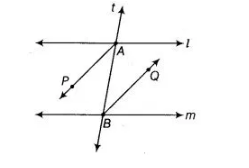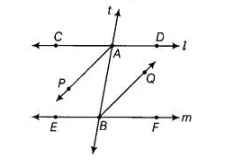# In the given figure, bisectors AP and BQ`
Question:

In the given figure, bisectors AP and BQ of the alternate interior angles are parallel, then show that l ||m.Solution:

Given, In the figure  AP|| BQ, AP and BQ are the bisectors of alternate interior angles ∠CAB and ∠ABF.

To show l || m

Proof Since, AP|| BQ and t is transversal, therefore ∠PAB = ∠ABQ

[alternate interior angles]

=> 2 ∠PAB = 2 ∠ABQ [multiplying both sides by 2]So, alternate interior angles are equal.

We know that, if two alternate interior angles are equal, then lines are parallel. Hence, l || m.